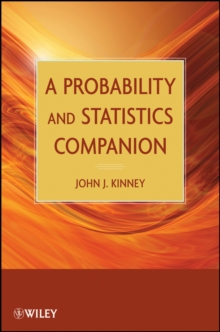Supporting your high street Find out how »
• My AccountA Probability and Statistics Companion Paperback / softback

Description

An accessible and engaging introduction to the study of probability and statistics Utilizing entertaining real-world examples, A Probability and Statistics Companion provides aunique, interesting, and accessible introduction to probability and statistics.

This one-of-a-kind book delves into practical topics that are crucial in the analysis of sample surveys and experimentation.

This handy book contains introductory explanations of the major topics in probability and statistics, including hypothesis testing and regression, while also delving into more advanced topics such as the analysis of sample surveys, analysis of experimental data, and statistical process control.

The book recognizes that there are many sampling techniques that can actually improve on simple random sampling, and in addition, an introduction to the design of experiments is provided to reflect recent advances in conducting scientific experiments.

This blend of coverage results in the development of a deeper understanding and solid foundation for the study of probability and statistics. Additional topical coverage includes: Probability and sample spaces Choosing the best candidate Acceptance sampling Conditional probability Random variables and discrete probability distributions Waiting time problems Continuous probability distributions Statistical inference Nonparametric methods Least squares and medians Recursions and probability Each chapter contains exercises and explorations for readers who wish to conduct independent projects or investigations.

The discussion of most methods is complemented with applications to engaging, real-world scenarios such as winning speeds at the Indianapolis 500 and predicting winners of the World Series.

In addition, the book enhances the visual nature of the subject with numerous multidimensional graphical representations of the presented examples. A Probability and Statistics Companion is an excellent book for introductory probability and statistics courses at the undergraduate level.

It is also a valuable reference for professionals who use statistical concepts to make informed decisions in their day-to-day work.

Information

• Format: Paperback / softback
• Pages: 280 pages
• Publisher: John Wiley & Sons Inc
• Publication Date:
• Category: Probability & statistics
• ISBN: 9780470471951

£71.95

£60.89

on all orders

Pick up orders

from local bookshops

£39.50

£20.50

£111.00

£98.75